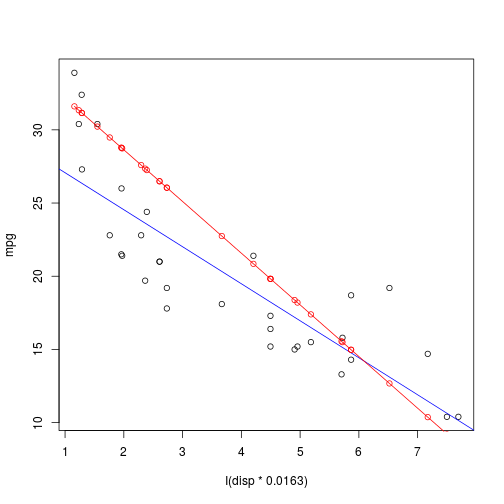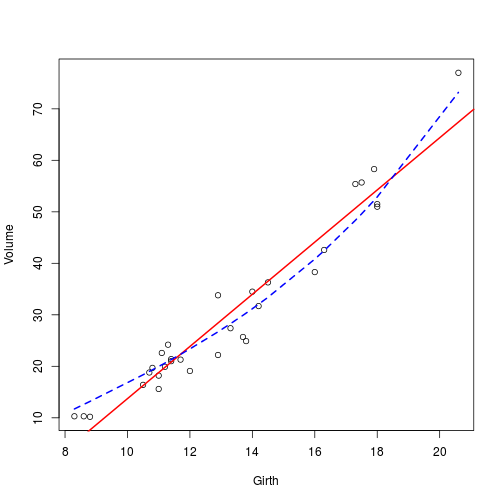Transponster

# Growing up

I’ve been playing with implementations of linear and logistic regression over the last couple of months, following the exercises from a machine learning course that I have been doing. So far I have been writing things in a very functional way, constantly defining specific functions to do what are essentially generic things.

I’ve also started to write a couple of my own packages, one of which I have published on github and zenodo. This one provides access to an API which allows users to download data collected by GPS collars on cattle. This package is entirely written as functions.

So, I think that it is about time that I started to mature my R programming career, and start to write in classes. I’ve been following the excellent guide hosted on cran, by Friedrich Leisch, and in this post, I’m going to re-factor some of my previously functional code for linear regression with regularisation by implementing it with classes, and the usual model related methods like print(), summary(), and predict().

## Before

So what does it look like at present? Rather than using the normal equation, this code uses the function optim to solve the problem:

optim takes in two functions, the cost function $J_\theta$ (J):

and a gradient function (gR):

These are taken as inputs in to the optim function:

And calling this, we get:

Just to check, I compare this with a simple linear regression with lm().

So the coefficients are not the same, but are pretty close nonetheless.

## Converting to S3 classes and methods

### Model function

As mentioned, I’m following this guide pretty closely, as it does exactly what I want to do. So I will start by producing a function to wrap up the optim call. optim must take inputs of functions for the cost and gradient, and so there is not too much that can be done to these functions as far as I can see, so for the moment I will leave them be.

For now, x must be specified as the usual $\mathbb{R}^{m \times n+1}$ matrix consisting of a column of 1s which correspond to the intercept.

So far so good. Now define the function vlrr() and the default method for it:

### vlrr class and default method

So now if I call call vlrr or the vlrr.default function, I should get the same right?

And because I defined the output in a standard way, I can use the standard methods to interrogate it:

I won’t print(model) as at present is will print all the slots in turn:

This ends up being very long, so it would be good to write a print method that returns something a bit shorter.

Better.

### Summary method

Model functions also usually have a summary method which prints out a nice summary of the model (e.g. summary.lm). For now I’ll just copy the default method given by Leisch, plus additional information about optim’s convergence success.

This is a bit messy, but we can define a print method for summary using the Coefmat function to order things nicely in the coefficients table.

Great, so this looks a lot more like the output we would expect from lm(), plus we have some statistics on the likelihood that our coefficients are different from zero.

### Formula method

It’s a bit tiresome having to specify x= and y= when in lm() and other models, we are able to specify a formula. In addition, if we want to include interaction terms $x_1 \times x_2$ for instance, this would need to be done in the input matrix x. By implementing a formula method, this can all be automated. This also gets us off the hook with having to cbind(1,x).

At present I must do:

But after implementing the formula method…

…things get much much simpler!

### Predict method

The last method I want to implement is predict as this makes it much easier to use the model once fit. Again I’m relying on Leisch here.

## Testing it out

So finally we can take this model and apply it to some other data. Here I take the model I built on fuel consumption from the mpg dataset, and use it to predict displacement values (disp) from the mtcars dataset. Note that displacement is given in cubic inches in mtcars but litres in mpg so needs to be multiplied by 0.0163 to put it on the same scale.## Writing a package

So the obvious thing to do now that this has all been done, is to wrap everything up into a package. I’m not going to go through the steps to do that here because it is a little bit long winded, and much better covered in the Leisch guide, and here by Hadley Wickham.

It’s a pretty simple process, and actually the most onerous (and arguably the most important) thing is to properly document all the functions. So I have put in a little bit of time, and produced the package vlrr, which is now available from a github repo.

This can be installed direct from within R using devtools::install_github("ivyleavedtoadflax/vlrr"). The library can then be called and run like any other.